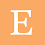### Mensuration Formulas

Mensuration        formulae
அளவையியல்      சூத்திரங்கள்

 Area of circle Area of circle = Π r ² Circumference of circle = 2 Π r Area of triangle Area of Equilateral-triangle = (√3/4) a² Perimeter of Equilateral-triangle = 3a Area of scalene triangle Area of scalene triangle = √s(s-a)(s-b)(s-c) Perimeter of scalene triangle  = a + b +  c Area of semicircle Area of Semi circle= (1/2) Π r² Perimeter of semi-circle = Πr Area of quadrant Area of quadrant = (1/4) Π r² Area of rectangle Area of rectangle = L x W Perimeter of rectangle=2(l+w) Area of square Area of square = a² Perimeter of square = 4a Area of parallelogram Area of parallelogram = b x h Area of quadrilateral Area of quadrilateral=(1/2) x d x (h₁+h₂) Area of rhombus Area of rhombus =(1/2) x (d₁ x d₂) Area of trapezoid Area of trapezoid =(1/2) (a + b) x h Area of sector Area of the sector = (θ/360) x Π r ² square units (or)  Area of the sector = (1/2) x l r square units    Length of arc = (θ/360) x 2Πr

 Cylinder Curved surface area = 2 Π r h Total Curved surface area = 2 Π r (h+r) Volume = Π r²h Cone Curved surface area = Π r l Total Curved surface area = Π r (L+r) Volume = (1/3)Π r²h L² = r² + h² Sphere Curved surface area = 4Π r² Volume = (4/3)Π r³ Hemisphere Curved surface area = 2Π r² Total Curved surface area=3Π r² Volume = (2/3)Π r³ Cuboid Curved surface area=4h(l+b) Total surface area = 2(lb+bh+h l) Volume = l x b x h Cube Curved surface area=4a² Total surface area = 6a² Volume = a³

Share:

1.This comment has been removed by the author.

2.Many students find mensuration topics very difficult as students need to understand various shapes, how the shape turns into different shapes when cut from a different angle, and formulas to solve a particular mensuration-related problem. The formulas you shared here would be very helpful for the students and if they learn them well then definitely they will be able to solve many questions. Mensuration is a very important topic when it comes to the geometry of the universe. By definition, mensuration refers to the part of geometry concerned with ascertaining lengths, areas, and volumes. Hence, it is easy to see why mensuration in learning maths is instrumental and plays a big part in real-world problems. Thanks for sharing.